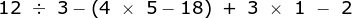Test Prep Plan - Take a practice test

Take this practice test to check your existing knowledge of the course material. We'll review your answers and create a Test Prep Plan for you based on your results.
How Test Prep Plans work
1
2Based on your results, we'll create a customized Test Prep Plan just for you!
3Study smarter
Study more effectively: skip concepts you already know and focus on what you still need to learn.

Saxon Algebra 2 Homeschool: Online Textbook Help Final Exam

Free Practice Test Instructions:

Choose your answer to the question and click 'Continue' to see how you did. Then click 'Next Question' to answer the next question. When you have completed the free practice test, click 'View Results' to see your results. Good luck!

Question 2 2. What pictured shape do we draw to identify the measured length of a triangle's side?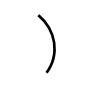Question 4 4. In the following right triangle, what is the equation for L as a function of x?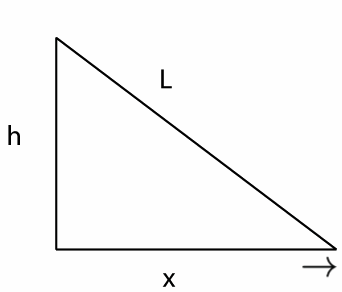Question 5 5. Find the solution to the equation below.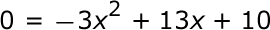Question 6 6. Use the quadratic formula to find the solutions to the equation below.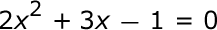Question 7 7. Simplify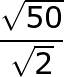Question 10 10. Simplify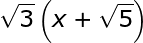Question 13 13. Solve